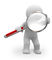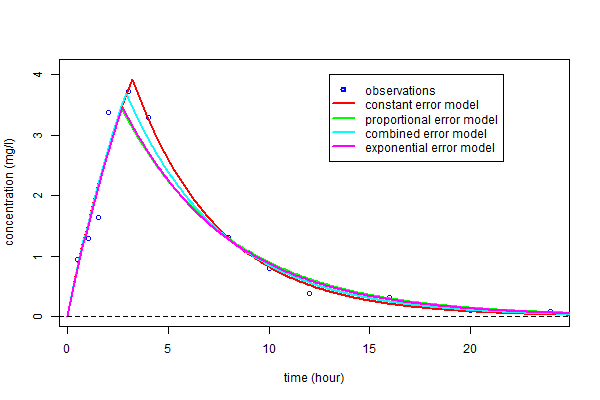# TestMarc2

## Introduction

A model built for real-world applications can involve various types of variable, such as measurements, individual and population parameters, covariates, design, etc. The model allows us to represent relationships between these variables.

If we consider things from a probabilistic point of view, some of the variables will be random, so the model becomes a probabilistic one, representing the joint distribution of these random variables.

Defining a model therefore means defining a joint distribution. The hierarchical structure of the model will then allow it to be decomposed into submodels, i.e., the joint distribution decomposed into a product of conditional distributions.

Tasks such as estimation, model selection, simulation and optimization can then be expressed as specific ways of using this probability distribution.

- A model is a joint probability distribution.

- A submodel is a conditional distribution derived from this joint distribution.

- A task is a specific use of this distribution.

We will illustrate this approach starting with a very simple example that we will gradually make more sophisticated. Then we will see in various situations what can be defined as the model and what its inputs are.

## An illustrative example

### A model for the observations of a single individual

Let $y=(y_j, 1\leq j \leq n)$ be a vector of observations obtained at times $t=(t_j, 1\leq j \leq n)$. We consider that the $y_j$ are random variables and we denote $p_y$ the distribution (or pdf) of $y$. If we assume a parametric model, then there exists a vector of parameters $\psi$ that completely define $y$.

We can then explicitly represent this dependency with respect to ${\bf \psi}$ by writing $p_y( \, \cdot \, ; \psi)$ for the pdf of $y$.

If we wish to be even more precise, we can even make it clear that this distribution is defined for a given design, i.e., a given vector of times $t$, and write $p_y(\, \cdot \, ; \psi, t)$ instead.

By convention, the variables which are before the symbol ";" are random variables. Those that are after the ";" are non-random parameters or variables. When there is no risk of confusion, the non-random terms can be left out of the notation.

-In this context, the model is the distribution of the observations $p_y(\, \cdot \, ; \psi, t)$.
-The inputs of the model are the parameters $\psi$ and the design $t$.

Example:

500 mg of a drug is given by intravenous bolus to a patient at time 0. We assume that the evolution of the plasmatic concentration of the drug over time is described by the pharmacokinetic (PK) model

$$f(t;V,k) = \displaystyle{ \frac{500}{V} }e^{-k \, t} ,$$

where $V$ is the volume of distribution and $k$ the elimination rate constant. The concentration is measured at times $(t_j, 1\leq j \leq n)$ with additive residual errors:

$$y_j = f(t_j;V,k) + e_j , \quad 1 \leq j \leq n .$$

Assuming that the residual errors $(e_j)$ are independent and normally distributed with constant variance $a^2$, the observed values $(y_j)$ are also independent random variables and

 $$y_j \sim {\cal N} \left( f(t_j ; V,k) , a^2 \right), \quad 1 \leq j \leq n.$$ (1.4)

Here, the vector of parameters $\psi$ is $(V,k,a)$. $V$ and $k$ are the PK parameters for the structural PK model and $a$ the residual error parameter. As the $y_j$ are independent, the joint distribution of $y$ is the product of their marginal distributions:

$$p(y ; \psi, t) = \prod_{j=1}^n p_j(y_j ; \psi,t_j) ,$$

where $p_j$ is the normal distribution defined in (1.4).Example:

500 mg of a drug is given by intravenous bolus to a patient at time 0. We assume that the evolution of the plasmatic concentration of the drug over time is described by the pharmacokinetic (PK) model

$$f(t;V,k) = \displaystyle{ \frac{500}{V} }e^{-k \, t} ,$$

where $V$ is the volume of distribution and $k$ the elimination rate constant. The concentration is measured at times $(t_j, 1\leq j \leq n)$ with additive residual errors:

$$y_j = f(t_j;V,k) + e_j , \quad 1 \leq j \leq n .$$

Assuming that the residual errors $(e_j)$ are independent and normally distributed with constant variance $a^2$, the observed values $(y_j)$ are also independent random variables and

 $$y_j \sim {\cal N} \left( f(t_j ; V,k) , a^2 \right), \quad 1 \leq j \leq n.$$ (1.4)

Here, the vector of parameters $\psi$ is $(V,k,a)$. $V$ and $k$ are the PK parameters for the structural PK model and $a$ the residual error parameter. As the $y_j$ are independent, the joint distribution of $y$ is the product of their marginal distributions:

$$p(y ; \psi, t) = \prod_{j=1}^n p_j(y_j ; \psi,t_j) ,$$

where $p_j$ is the normal distribution defined in (1.4).Example:

500 mg of a drug is given by intravenous bolus to a patient at time 0. We assume that the evolution of the plasmatic concentration of the drug over time is described by the pharmacokinetic (PK) model

$$f(t;V,k) = \displaystyle{ \frac{500}{V} }e^{-k \, t} ,$$

where $V$ is the volume of distribution and $k$ the elimination rate constant. The concentration is measured at times $(t_j, 1\leq j \leq n)$ with additive residual errors:

$$y_j = f(t_j;V,k) + e_j , \quad 1 \leq j \leq n .$$

Assuming that the residual errors $(e_j)$ are independent and normally distributed with constant variance $a^2$, the observed values $(y_j)$ are also independent random variables and

 $$y_j \sim {\cal N} \left( f(t_j ; V,k) , a^2 \right), \quad 1 \leq j \leq n.$$ (1.4)

Here, the vector of parameters $\psi$ is $(V,k,a)$. $V$ and $k$ are the PK parameters for the structural PK model and $a$ the residual error parameter. As the $y_j$ are independent, the joint distribution of $y$ is the product of their marginal distributions:

$$p(y ; \psi, t) = \prod_{j=1}^n p_j(y_j ; \psi,t_j) ,$$

where $p_j$ is the normal distribution defined in (1.4).

### A model for several individuals

Now let us move to $N$ individuals. It is natural to suppose that each is represented by the same basic parametric model, but not necessarily the exact same parameter values. Thus, individual $i$ has parameters $\psi_i$. If we consider that individuals are randomly selected from the population, then we can treat the $\psi_i$ as if they were random vectors. As both ${\bf y}=(y_i , 1\leq i \leq N)$ and ${\bf \psi}=(\psi_i , 1\leq i \leq N)$ are random, the model is now a joint distribution: $p_ypsi$. Using basic probability, this can be written as:

$${\mathrm p}({\bf y},{\bf \psi}) = \mathrm{p}({\bf y} | {\bf \psi}) \, \mathrm{p}({\bf \psi}) .$$

If $p_\psi$ is a parametric distribution that depends on a vector $\theta$ of population parameters and a set of individual covariates ${\bf c}=(c_i , 1\leq i \leq N)$, this dependence can be made explicit by writing $p_\psi(\, \cdot \,;\theta,{\bf c})$ for the pdf of ${\bf \psi}$. Each $i$ has a potentially unique set of times $t_i=(t_{i1},\ldots,t_{i \ \!\!n_i})$ in the design, and $n_i$ can be different for each individual.

- In this context, the model is the joint distribution of the observations and the individual parameters:

$${\mathrm p}({\bf y} , {\bf \psi}; \theta, {\bf c},{\bf t})= {\mathrm p}({\bf y} , {\bf \psi};{\bf t}) \, \mathrm{p}({\bf \psi};\theta,{\bf c}) .$$

- The inputs of the model are the population parameters $\theta$, the individual covariates ${\bf c}=(c_i , 1\leq i \leq N)$ and the measurement times

${\bf t}=(t_{ij} ,\ 1\leq i \leq N ,\ 1\leq j \leq n_i)$.</div> </div> <div class="noprint" style="float:none; border-left: 14px solid #DABDAB; border-radius: 0.5em 0.5em 0.5em 0.5em; background-color:beige; padding:12px; margin-left: 2%; margin-right: 8%;"> <div style="text-align: left; padding-left: 0.5em; padding-top:0.5em; font-size:13pt;font-family:Segoe UI;font-weight:bold">Remarks</div><br> <div style="text-align: left; padding-left: 1.2em; padding-bottom:0.7em">Approximating the fraction $\hat{\psi}/\widehat{\rm s.e}(\hat{\psi}_k)$ by the normal distribution is a "good" approximation only when the number of observations $n$ is large. A better approximation should be used for small $n$. In the model $y_j = f(t_j ; \phi) + a\varepsilon_j$, the distribution of $\hat{a}^2$ can be approximated by a chi-square distribution with $(n-d_\phi)$ degrees of freedom, where $d_\phi$ is the dimension of $\phi$. The quantiles of the normal distribution can then be replaced by those of a Student's $t$-distribution with $(n-d_\phi)$ degrees of freedom.</div> </div> <br> =='"UNIQ--h-4--QINU"' Examples With Equations/Code/Tables == <div class="noprint" style="border-left: 6px solid #C04000; margin-left:4%; margin-right:5%;padding-top:0.8em"> [[Image:man02.jpg|52px|left|top|link=]] <div style="text-align: left; padding-left: 2.5em; font-size:13pt; font-weight:bold"><u>Example 1:</u><span style="font-size:12pt;font-weight:normal"> Poisson model with time varying intensity</span></div> <br> <div style="text-align: left; padding-left: 3%"></div> {| cellspacing="15" cellpadding="15" | style="width:40%" | <div style="text-align: left;font-size:12pt;padding-left:1%;padding-right:2%">$$\begin{array}{c} \psi_i &=& (\alpha_i,\beta_i) \\[0.3cm] \lambda(t,\psi_i) &=& \alpha_i + \beta_i\,t \\[0.3cm] \mathbb{P}(y_{ij}=k) &=& \displaystyle{ \frac{\lambda(t_{ij} , \psi_i)^k}{k!} } e^{-\lambda(t_{ij} , \psi_i)}\\ \end{array}$$</div> | style="width:50%" | <div class="noprint" style="background-color:#EFEFEF; border: 1px solid darkgray; border-radius:1em"> <div style="margin-top:1em;margin-left:1em">[[Image:monolix_icon2.png|left|top|link=]]</div> <div style="text-align: left; padding-left: 4em; margin-top:0.5em; font-family:'courier new'; font-size:14pt; font-weight:bold; color: #0095B6; margin-top:1.2em">MLXTran <span style="font-weight:normal; font-size:13pt"></span></div> <div style="text-align: left; padding-left: 1.2em; font-family:'courier new';margin-bottom:1em">'"UNIQ--pre-00000000-QINU"'</div> </div> |} </div> [[:Template:ExampleWithTable1bis]] <div class="noprint" style="margin-left: 2em; border-left: 6px solid #F4C430; marging-left:3.5%; margin-right:3.5%;padding-top:0.8em"> [[Image:Icone4.jpg|50px|left|top]] <div style="text-align: left; padding-left: 2.5em; font-size:14pt"><u>'''Example 1:'''</u><span style="font-size:12pt"> Poisson model with time varying intensity</span></div> <br> <div style="text-align: left; padding-left: 2.5em; font-size:12pt;"></div> {| cellspacing="5" cellpadding="5" | style="width:550px;" | <div style="text-align: left;font-size: 12pt;padding-left:1em;font-family:arial;">$$\begin{array}{c} \psi_i &=& (\alpha_i,\beta_i) \\[0.3cm] \lambda(t,\psi_i) &=& \alpha_i + \beta_i\,t \\[0.3cm] \mathbb{P}(y_{ij}=k) &=& \displaystyle{ \frac{\lambda(t_{ij} , \psi_i)^k}{k!} } e^{-\lambda(t_{ij} , \psi_i)}\\ \end{array}$$</div> | style="width:550px;" | <div class="noprint" style="background-color:#EFEFEF; border: 1px solid darkgray; border-radius:1em"> <div style="margin-top:1em;margin-left:1em">[[Image:monolix_icon2.png|left|top|link=]]</div> <div style="text-align: left; padding-left: 4em; margin-top:0.5em; font-family:'courier new'; font-size:14pt; font-weight:bold; color: #0095B6; margin-top:1.2em">MLXTran <span style="font-weight:normal; font-size:13pt"></span></div> <div style="text-align: left; padding-left: 1.2em; font-family:'courier new';margin-bottom:1em">'"UNIQ--pre-00000001-QINU"'</div> </div> |} </div> [[:Template:ExampleWithTable 4]] {| cellpadding="5" cellspacing="5" | style="width:550px;" | <div class="noprint" style="background-color:#EFEFEF; border: 1px solid darkgray; border-radius:1em; margin-left:1%; margin-right:1%"> :<div style="margin-top:1em">[[Image:Rstudio.png|33px|left|top]]</div> <div style="text-align: left; padding-left: 4em; font-family:'courier new'; font-size:14pt; font-weight:bold; color: #007FFF; margin-top:1em">R <span style="font-size:13pt; font-weight:normal"> </span></div><br> <div style="text-align: left; padding-left: 1.2em; font-family:'courier new';margin-bottom:1em">'"UNIQ--pre-00000002-QINU"'</div> </div> | style="width:550px;" | :Here are the parameter estimation results: <div class="noprint" style="background-color:#EFEFEF; border: 1px solid darkgray; border-radius:1em; margin-left:1%; margin-right:1%"> <div style="text-align: left; margin-left: 1.1em; font-family:'courier new'; color:blue;margin-top:0.9em; margin-bottom:0.9em">'"UNIQ--pre-00000003-QINU"'</div> </div> |} =='"UNIQ--h-5--QINU"' Equations == Here are some examples of these various types of data: * Continuous data with a normal distribution: <div class="noprint" style="float:none; border:1px solid #50C878;border-radius:1em; background-color:#ECFCF4;padding:14px;margin-left: 25%; margin-right: 25%"> <div style="text-align:center; font-size:13pt"> $$y_{ij} \sim {\cal N}\left(f(t_{ij},\psi_i),\, g^2(t_{ij},\psi_i)\right)$$ </div> </div> :Here, $\lambda(t_{ij},\psi_i)=\left(f(t_{ij},\psi_i),\,g(t_{ij},\psi_i)\right)$, where $f(t_{ij},\psi_i)$ is the mean and $g(t_{ij},\psi_i)$ the standard deviation of $y_{ij}$. * Categorical data with a Bernoulli distribution: <div class="noprint" style="float:none; border:1px solid #50C878;border-radius:1em; background-color:#ECFCF4;padding:14px;margin-left: 25%; margin-right: 25%"> <div style="text-align:center; font-size:13pt">$$y_{ij} \sim {\cal B}\left(\lambda(t_{ij},\psi_i)\right)$$ </div> </div> :Here, $\lambda(t_{ij},\psi_i)$ is the probability that $y_{ij}$ takes the value 1.

 $$y_{ij} \sim {\cal N}\left(f(t_{ij},\psi_i),\, g^2(t_{ij},\psi_i)\right)$$ (2.1)

$$y_{ij} \sim {\cal N}\left(f(t_{ij},\psi_i),\, g^2(t_{ij},\psi_i)\right)$$

$${\cal L}(\theta ; \psi_1,\psi_2,\ldots, \psi_N) \ \ = \ \ \prod_{i=1}^{N}{\mathrm p}(\psi_i ; c_i , \theta).$$This is the caption of the figure